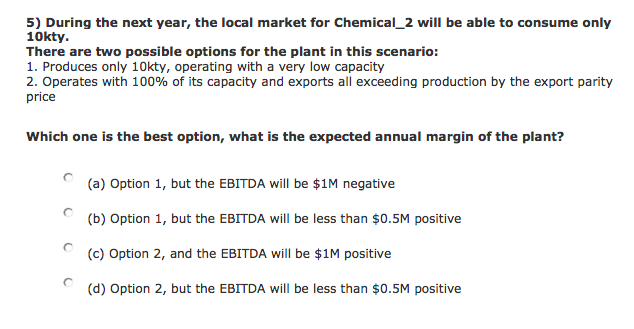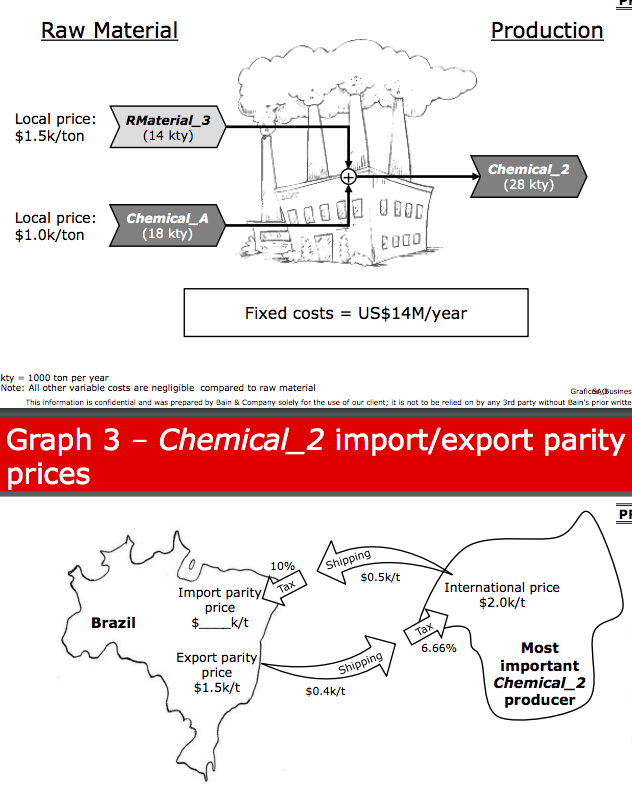New answer on Jan 26, 2021
1.4 k Views

Hi,

This is a question from Bain sample case. I attach the question and relevant graphs below. Would appreciate if someone can give me hints on how to solve this problem. Thank you very much!• Date ascending
• Date descending

Hi there,

You need to calculate the import parity price from the last exhibit (2.0 + .5 times 10%)

Then, calculate the total costs of production (material 1 and 2) from the 1st exhibit

Finally, look at the amount of product produced, the total cost for it, and the total revenue to get your answer!

Hello!

Could you share where you obtained this?

Cheers,

Clara

Yes